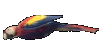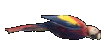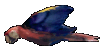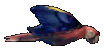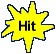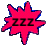Solve Equations in 2 Steps: y+a=b, a+y=b, y-a=b,  ay = b,  y/a = b     Math Fun Game Tips:

- An equation has an equal sign '=' between its left side and right side.   Example: y+7=19
Finding the answer to an equation, is called solving the equation.
To solve y+7=19, you need to isolate the variable y by itself on one side of the equation.

- For an equation y+7=19, the answer y=12 is the value of the variable y that satisfies the equation.
To satisfy an equation means to make its left side value equal its right side value.
When you check the answer y=12, the left side value of y+7 evaluates as 12+7 = 19.
When you check the right side value of y+7=19, it is already 19 with no evaluation work needed.

The answer y=12 that satisfies the equation y+7=19 can be called the solution or root of the equation.

- When solving an equation, you treat both its left side and its right side equally.
For eg:  if you subtract 7 from the left side of y+7=19, then you also subtract 7 from the right side.
To solve y+7=19, a complete solution includes the step y+7-7=19-7 before writing the final step y=12.
Notice that to remove +7 from y+7, you use the inverse of addition, namely subtraction.

- Similarly, the standard method for solving an equation such as w-8 = 20 to find the value of w
is to use the step w-8+8 = 20+8 before stating the final answer w=28.
Notice that to remove -8 from w-8, you use the inverse of subtracting 8, namely adding 8 to each side.

- The standard method for solving an equation such as 6y=42 to find the value of y
is to show the step 6y/6 = 42/6 before stating the final answer y=7.
Notice that to remove the 6 from 6y, you use the inverse of multiplication by 6, namely division by 6.

- Also, the standard method for solving an equation such as w/8 = 3 to find the value of w
is to show the step (w/8)x8 = 3x8 before stating the final answer w=24.
Notice that to remove the 8 from w/8, you use the inverse of dividing by 8, namely multiplying by 8.

- Your Game Score is reduced by the number of parrot hits.

- To slow the game speed repeat tap/click on the word Slider.
- To increase the game speed repeat tap/click on the word Math.
- Speed can also be adjusted with a keyboard's - and + keys.

- Refresh/Reload the web page to restart the game.